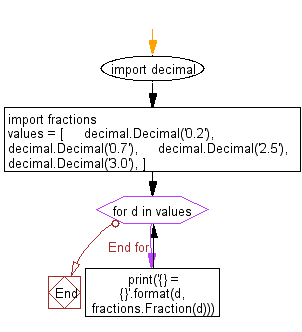﻿ Python Math: Create the fraction instances of decimal numbers - w3resource# Python Math: Create the fraction instances of decimal numbers

## Python Math: Exercise-45 with Solution

Write a Python program to create the fraction instances of decimal numbers.

Sample Solution:-

Python Code:

``````import decimal
import fractions
values = [
decimal.Decimal('0.2'),
decimal.Decimal('0.7'),
decimal.Decimal('2.5'),
decimal.Decimal('3.0'),
]

for d in values:
print('{} = {}'.format(d, fractions.Fraction(d)))
```
```

Sample Output:

```0.2 = 1/5

0.7 = 7/10

2.5 = 5/2

3.0 = 3
```

Flowchart:Python Code Editor:

Have another way to solve this solution? Contribute your code (and comments) through Disqus.

What is the difficulty level of this exercise?

Test your Python skills with w3resource's quiz

﻿

## Python: Tips of the Day

Python: Get the Key Whose Value Is Maximal in a Dictionary

```>>> model_scores = {'model_a': 100, 'model_z': 198, 'model_t': 150}
>>> # workaround
>>> keys, values = list(model_scores.keys()), list(model_scores.values())
>>> keys[values.index(max(values))]
'model_z'
>>> # one-line
>>> max(model_scores, key=model_scores.get)
'model_z'
```